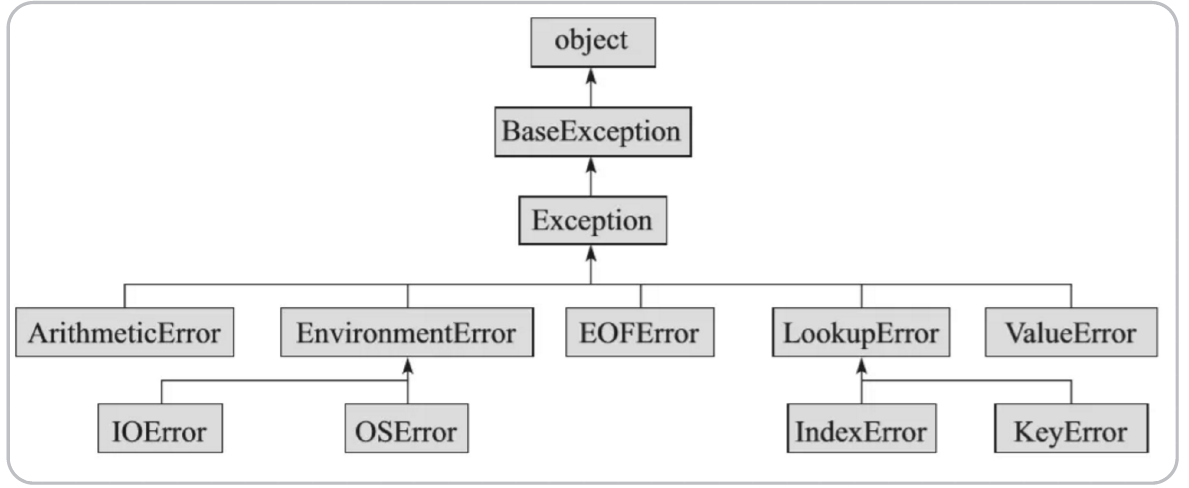# Python中的基本概念：变量、运算符与数据类型

Python中的注释分为单行和多行注释，分别用#’’’’’’或者””””””表示——

# 单行注释
print("Hello World!")

'''

'''
print("Hello China!")

'''

'''

print(5%2)

print(2**3)

print(11//3)



'''

'''

print("按位取反：",~0)

print("按位与操作：",1&1)

print("按位或操作：",1|0)

print("按位异或操作：",1^1)

print("按位左移3位：",11 << 3)

print("按位右移2位：",11 >> 2)

# 三元运算符

x,y = 4,5

print(x if x < y else y)

'''
'''
is, is not 对比的是两个变量的内存地址

==, != 对比的是两个变量的值

-假如比较的两个变量，指向的都是地址不可变的类型（str等），那么is，is not 和 ==，！= 是完全等价的。

-假如对比的两个变量，指向的是地址可变的类型（list，dict，tuple等），则两者是有区别的

'''

a = ["Hello"]

b = ["Hello"]

print(a is b, a == b)

a = "Hello"

b = "Hello"

print(a is b, a == b)

1、一元运算符优先于二元运算符；

2、先算术运算，后移位运算，最后位运算，例如1<<3+2&7等价于(1<<(3+2))&7;

3、逻辑运算最后结合起来。

Python中的变量在使用之前需要进行赋值操作，同时对变量的取名有一定的要求：可以包含字母、数字、下划线等，但是不能以数字开头，不然无法辨认，而且对于字母的大小写是敏感的。

Python 里面有很多用途广泛的包 (package)，用什么你就引进 (import) 什么。包也是对象，也可以用上面提到的dir(decimal) 来看其属性和方法。比如 getcontext() 显示了 Decimal 对象的默认精度值是 28 位 ( prec=28 )。

# 找到一个整数的二进制表示，再返回其长度

a = 1031

print(bin(a))

print(a.bit_length())

import decimal

from decimal import Decimal

a = decimal.getcontext()

print(a)

# 用getcontext().prec来调整精度

decimal.getcontext().prec = 4

c = Decimal(1)/Decimal(3)

print(c)

1. 基本类型：整型、浮点型、布尔型

2. 容器类型：字符、元组、列表、字典和集合

bool 作用在基本类型变量：X 只要不是整型 0、浮点型 0.0bool(X) 就是 True ，其余就是 False

bool 作用在容器类型变量：X 只要不是空的变量，bool(X) 就是 True ，其余就是 False

1. 对于数值变量，0 , 0.0 都可认为是空的。

2. 对于容器变量，里面没元素就是空的。

# bool判断不同类型的变量，空就是False,否则就是true

print(type(True),bool(True),bool(False))

print(type(()),bool(()),bool((10,)))

print(type(5.2))

# 获取类型信息

print(type(1))

print(type(True))

print(isinstance('5.2',str))

print(isinstance(True,bool))

1. type() 不会认为子类是一种父类类型，不考虑继承关系。

2. isinstance() 会认为子类是一种父类类型，考虑继承关系。

Python中的数据类型转换，很直接地可以用类似于int(x)float(y)等进行转换。

print(*objects, sep=' ', end='\n', file=sys.stdout, flush=False)

1. 将对象以字符串表示的方式格式化输出到流文件对象file里。其中所有非关键字参数都按str() 方式进行转换为字符串输出；

2. 关键字参数sep 是实现分隔符，比如多个参数输出时想要输出中间的分隔字符；

3. 关键字参数end 是输出结束时的字符，默认是换行符\n

4. 关键字参数file 是定义流输出的文件，可以是标准的系统输出sys.stdout ，也可以重定义为别的文件；

5. 关键字参数flush 是立即把内容输出到流文件，不作缓存。

# 位运算

n << 1 -> 计算 n*2

n >> 1 -> 计算 n//2，负奇数的运算不可用

n << m -> 计算 n*(2^m)，即乘以 2 m 次方

n >> m -> 计算 n//(2^m)，即除以 2 m 次方

1 << n -> 2^n

a ^= b

b ^= a

a ^= b

00 00 11 10 -> 14

&

11 11 00 10 -> -14

a | (1<<i) -> i 插入到集合中

a & ~(1<<i) -> i 从集合中删除

a & (1<<i) -> 判断 i 是否属于该集合（零不属于，非零属于）

a -> ~a

a b -> a & b

a b -> a | b

a b -> a & (~b)

# 利用位运算进行整数集合

print(bin(3))

print(bin(-3&0xffffffff))



Pythonbin 一个负数（十进制表示），输出的是它的原码的二进制表示加上个负号，巨坑。

2. Python中的整型是补码形式存储的。

3. Python中整型是不限制长度的不会超范围溢出。

# 条件语句

if expression1:

expr1_true_suite

elif expression2:

expr2_true_suite

.

.

elif expressionN:

exprN_true_suite

else:

expr_false_suite

# 循环语句

Range()函数——range([start,] stop[, step=1])

1. 这个BIF（Built-in functions）有三个参数，其中用中括号括起来的两个表示这两个参数是可选的。

2. step=1 表示第三个参数的默认值是1。

3. range 这个BIF的作用是生成一个从start 参数的值开始到stop 参数的值结束的数字序列，该序列包含start 的值但不包含stop 的值。

enumerate()函数——enumerate(sequence, [start=0])

1. sequence -- 一个序列、迭代器或其他支持迭代对象。

2. start -- 下标起始位置。

3. 返回 enumerate(枚举) 对象

Break语句——break 语句可以跳出当前所在层的循环。

continue语句——continue 终止本轮循环并开始下一轮循环。

pass语句——pass 语句的意思是“不做任何事”，如果你在需要有语句的地方写任何语句，那么解释器会提示出错，而 pass 语句就是用来解决这些问题的。

(1)、列表推导式：[ expr for value in collection [if condition] ]

(2)、元组推导式：( expr for value in collection [if condition] )

(3)字典推导式：{ key_expr: value_expr for value in collection [if condition] }

(4)集合推导式：{ expr for value in collection [if condition] }

# range函数

print(range(2,9))

# 列表推导式

x = [-4,-2,0,2,4]

print([a*2 for a in x])

# 元组推导式

print(x for x in range(10))

# 字典推导式

b = {i: i % 2 == 0 for i in range(10) if i % 3 == 0}

print(b)

# 集合推导式

c = {i for i in [1, 2, 3, 4, 5, 5, 6, 4, 3, 2, 1]}

print(c)

# 异常处理try-except语句：

try:

except Exception[as reason]:

try 语句按照如下方式工作：

1. 首先，执行try 子句（在关键字try 和关键字except 之间的语句）

2. 如果没有异常发生，忽略except 子句， try 子句执行后结束。

3. 如果在执行try 子句的过程中发生了异常，那么try 子句余下的部分将被忽略。如果异常的类型和except 之后的名称相符，那么对应的except 子句将被执行。最后执行try 语句之后的代码。

4. 如果一个异常没有与任何的except 匹配，那么这个异常将会传递给上层的try 中。

# try-except语句

try:

int("abc")

s = 1 + '1'

f = open('test.txt')

f.close()

except OSError as error:

print('打开文件出错\n原因是：' + str(error))

except TypeError as error:

print('类型出错\n原因是：' + str(error))

except ValueError as error:

print('数值出错\n原因是：' + str(error))


try-except-else语句尝试查询不在dict 中的键值对，从而引发了异常。这一异常准确地说应属于KeyError ，但由

# try-except-else 语句

dict1 = {'a': 1, 'b': 2, 'v': 22}

try:

x = dict1['y']

except KeyError:

print('键错误')

except LookupError:

print('查询错误')

else:

print(x)



try-except-finally语句：

try:

except Exception[as reason]:

finally:

# try-except-finally语句

def divide(x, y):

try:

result = x / y

print("result is", result)

except ZeroDivisionError:

print("division by zero!")

finally:

print("executing finally clause")

divide(2, 1)

divide(2, 0)

divide("2", "1")

try-except-else语句

try:

except:

else:

try:

except(Exception1[, Exception2[,...ExceptionN]]]):

else:

# try-except-else语句

try:

fh = open("testfile", "w")

fh.write("这是一个测试文件，用于测试异常!!")

except IOError:

print("Error: 没有找到文件或读取文件失败")

else:

print("内容写入文件成功")

fh.close()



raise语句

Python 使用raise 语句抛出一个指定的异常。

# raise语句

try:

raise NameError('HiThere')

except NameError:

print('An exception flew by!')



12-153万+09-07635
09-06235
09-0732
01-201万+
08-2878
09-0617
09-0925
09-0636
12-1925
09-0536
12-2881
12-1935
09-0823
09-08144
09-061749
09-0741
06-198万+
08-209972